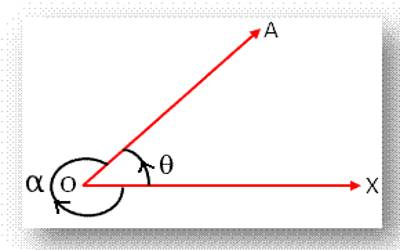The word ‘Trigonometry’ has been derived from Greek words ‘trigon’ meaning three angles and ‘metron’ which means measurement. The angles mπ/n (with m, n integers) for which the trigonometric functions may be expressed in terms of finite root extraction of real numbers are limited to values of m which are precisely those which produce constructible polygons.

Therefore, basically, the object of trigonometry was to deal with the measurement of triangles. Trigonometry was also involved in the establishment of relations between the sides, angles, and area of a triangle. Nowadays, the object of trigonometry deals not only with the angles of a triangle but the measure of all sorts of positive and negative angles. Thus this branch of mathematics has widened. Trigonometry is also used in science which involves measurement of angles.

Measure of Angles in Trigonometry

The concept of measure of angles in trigonometry is more general compared to a geometrical angle.

More than thousands of years ago, the ancient Babylonians chose 360 as their number to measure angles. An angle in geometry is supposed to be formed by the intersection of two lines and always varies from 0 to 360°. The unit of an angle is called a ‘degree’ (°). One full rotation indicates 360°.

An angle θ is said to be acute angle if 0° ≤ θ < 90°

An angle θ is said to be right angle if θ = 90°

An angle θ is said to be obtuse angle if 90° < θ < 180°

An angle θ is said to be straight angle if θ = 180°

An angle θ is said to be reflex angle if 180° < θ < 360°

Geometrical angles are always positive. In other words, in geometry, there is no use of negative angles. But the measure of angles in trigonometry is formed by the revolution of a straight line about a fixed point and the magnitude of such angle has no definite limit i.e., a trigonometrical angle may have any positive or negative value.Let OX be a fixed line on the plane of this page and OA be a revolving line whose initial position coincides with OX. If OA begins to revolve around O and comes from its initial position OX to the final position OA then we say that OA forms < XOA with OX. Here, ∠XOA is called a trigonometrical angle, O is its vertex, OX the initial arm and OA the final arm of the angle. If OA revolves about O in the anti-clockwise sense and starting from the initial position OX comes to the final position OA then ∠XOA = (θ) formed by the generating line OA is called a trigonometrical positive angle. Conversely, if the generating line OA revolves about O in the clockwise sense and starting from the initial position OX comes to the position OA then ∠XOA (=α) formed by OA is called a trigonometrical negative angle.

A trigonometrical angle may have any positive or negative value i.e., such an angle has no definite limit. To make the point clear we take a fixed point O on the plane of the paper and draw two mutually perpendicular lines XOX’ and YOY’ through O.Clearly, the drawn two lines divide the plane of the paper into four regions XOY , YOX‘, X ‘OY‘ and Y‘OX ; these four regions are respectively called the first, second, third and fourth quadrants. Now, assume that the generating line OA revolves about O in the anti-clockwise sense and starting from the initial position OX comes in the positions OA, OB, OC, OD describing angles ∠XOA, ∠XOB, ∠XOC and ∠XOD in the first, second, third and fourth quadrants respectively.

Clearly, each of the angles ∠XOA, ∠XOB, ∠XOC, ∠XOD is positive and 0 < ∠XOA < 90°, 90° < ∠XOB < 180°, 180° < ∠XOC < 270° and 270° < ∠XOD < 360°.

Thus, any positive angle between 0° and 360° can be described by the revolving line if it does not complete a complete revolution in the anti-clockwise sense and the angle 360° is described when it coincides with OX after a complete revolution. If OA revolves further in the same direction then an angle greater than 360° is described by it. Clearly, an angle between 360° and 720° is described by the revolving line OA if it completes one revolution but does not complete two revolutions in the anti-clockwise sense. In this way, a positive angle of any given magnitude can be described by OA by its repeated revolution in the anti-clockwise sense.

For example, consider the measure of angles in trigonometry 2770°. Since 2770° = 7 × 360° + 180° + 70°, hence, an angle of magnitude 2770° is described by the revolving line OA if it coincides with OC in the third quadrant after making seven complete revolutions in the anti-clockwise sense. Similarly, if the revolving line OA starts from the initial position OX and revolves about O in the clockwise sense, then the negative angle of any given magnitude can be described by OA.

Information Source: